## Powers and Exponents

Before you begin working with powers and exponents, some basic definitions are necessary.

An exponent is a positive or negative number or 0 placed above and to the right of a quantity. It expresses the power to which the quantity is to be raised or lowered. In 4 3, 3 is the exponent. It shows that 4 is to be used as a factor three times: 4 × 4 × 4 (multiplied by itself twice). 4 3 is read as four to the third power (or four cubed).

4 = 2 × 2 × 2 × 2 = 16

2 = 3 × 3 = 9

3 = 5 × 5 × 5 = 125

Remember: x 1 = x and x 0 = 1 when x is any number (other than 0).If the exponent is negative, such as 4 –2, the number and exponent may be dropped under the number 1 in a fraction to remove the negative sign.

Simplify the following by first removing the negative signs and then removing the exponents.

–2

–3

–4

–1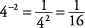Two specific types of powers should be noted: squares and cubes. To square a number, just multiply it by itself the way you'd find the area of a square (the exponent is 2). For example, 6 squared (written 6 2) is 6 × 6, or 36. 36 is called a perfect square (the square of a whole number). Following is a partial list of perfect squares:

2 = 1

2 = 4

2 = 9

2 = 16

2 = 25

2 = 36

2 = 49

2 = 64

2 = 81

10 2 = 100

11 2 = 121

12 2 = 144

To cube a number, just multiply it by itself twice the way you'd find the volume of a cube (the exponent is 3). For example, 5 cubed (written 5 3 is 5 × 5 × 5, or 125. 125 is called a perfect cube (the cube of a whole number). Following is a partial list of perfect cubes.

3 = 1 × 1 × 1 = 1

3 = 2 × 2 × 2 = 8

3 = 3 × 3 × 3 = 27

3 = 4 × 4 × 4 = 64

3 = 5 × 5 × 5 = 125

3 = 6 × 6 × 6 = 216

3 = 7 × 7 × 7 = 343

To multiply two numbers with exponents, if the base numbers are the same, simply keep the base number and add the exponents.

Multiply the following, leaving the answers with exponents.

3 × 2 5

2 × 3 5

4 × 5 7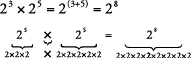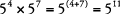To divide two numbers with exponents, if the base numbers are the same, simply keep the base number and subtract the second exponent from the first, or the exponent of the denominator from the exponent of the numerator.

Divide the following, leaving the answers with exponents.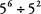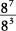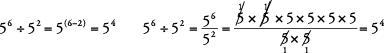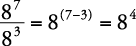To multiply or divide numbers with exponents, if the base numbers are different, you must simplify each number with an exponent first and then perform the operation.

Simplify and perform the operation indicated.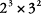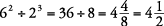For problems such as those in Example , some shortcuts are possible.

To add or subtract numbers with exponents, whether the base numbers are the same or different, you must simplify each number with an exponent first and then perform the indicated operation.

Simplify and perform the operation indicated.

2 – 2 3

2 + 3 3

2 + 9 3

3 – 2 2

2 – 2 3 = 9 – 8 = 1

2 + 3 3 = 25 + 27 = 52

2 + 9 3 = 16 + 729 = 745

3 – 2 2 = 8 – 4 = 4

If a number with an exponent is taken to another power (4 23, simply keep the original base number and multiply the exponents.

Multiply the following and leave the answers with exponents.

(6 32

(3 24

(5 43

(6 32 = 6 (3 × 2) = 6 6

(3 24 = 3 (2 × 4) = 3 8

(5 43 = 5 (4 × 3) = 5 12